trustle > EngliSea > Corona Safe Schooling > mathematics > Quick Math Index > 15 Geometry

A branch of mathematics concerned with questions of shape, size, relative position of figures, and the properties of space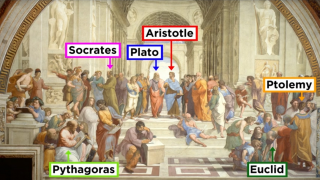01 Introduction to Geometry
If you could go back and visit any time in the history of mankind, which would it be?
5000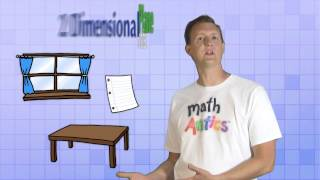01 Points, Lines, & Planes
Math Antics - Points, Lines, & Planes
5080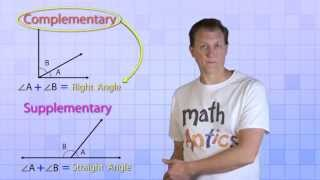02 Angle Basics
Math Antics - Angle Basics
5081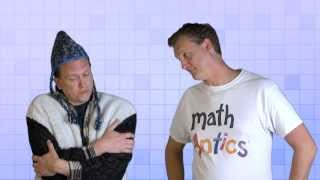03 Angles & Degrees
Math Antics - Angles & Degrees
5097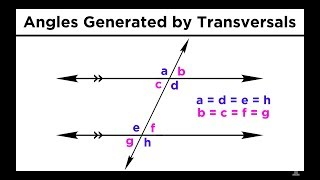03 Types of Angles and Angle Relationships
Types of Angles and Angle Relationships
500204 Polygons
Math Antics - Polygons
5101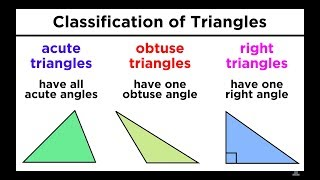04 Types of Triangles in Euclidian Geometry
Types of Triangles in Euclidian Geometry
5003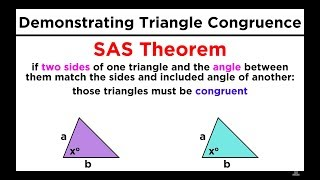05 Proving Triangle Congruence and Similarity
Proving Triangle Congruence and Similarity
5004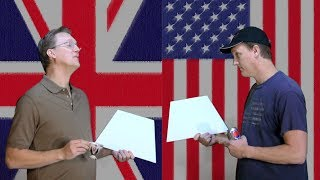06 Quadrilaterals
5157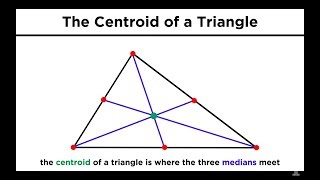06 Special Lines in Triangles: Bisectors, Medians, and Altitudes
Special Lines in Triangles: Bisectors, Medians, and Altitudes
5006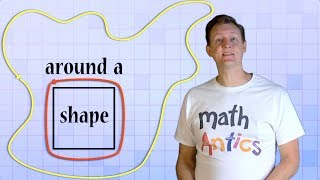07 Perimeter
Math Antics - Perimeter
5158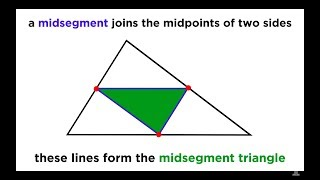07 The Triangle Midsegment Theorem
The Triangle Midsegment Theorem
5007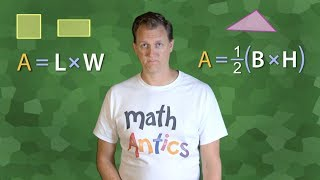08 Area
Math Antics - Area
5159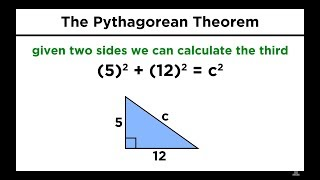08 The Pythagorean Theorem
The Pythagorean Theorem
5008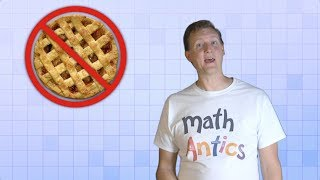09 Circles, What Is PI?
Math Antics - Circles, What Is PI?
5160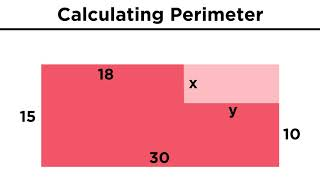10 Calculating the Perimeter of Polygons
Calculating the Perimeter of Polygons
5010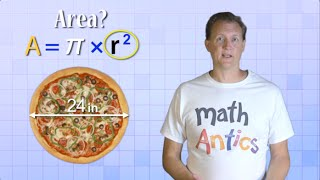10 Circles, Circumference And Area
Math Antics - Circles, Circumference And Area
5161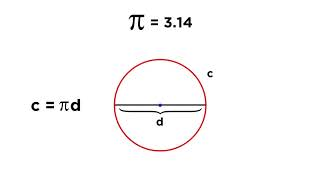11 Circles: Radius, Diameter, Chords, Circumference, and Sectors
Circles: Radius, Diameter, Chords, Circumference, and Sectors
5011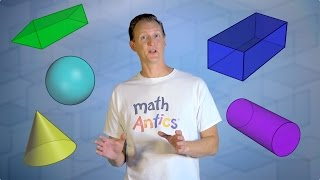11 Volume
Math Antics - Volume
5162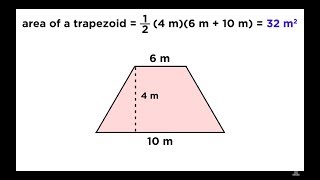12 Calculating the Area of Shapes
Calculating the Area of Shapes
5012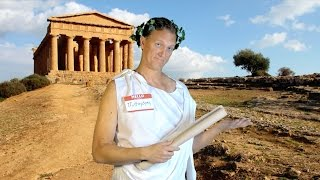12 The Pythagorean Theorem
Math Antics - The Pythagorean Theorem
5163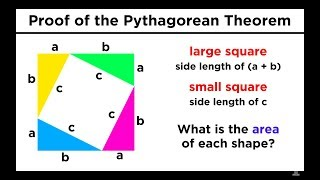13 Proving the Pythagorean Theorem
Proving the Pythagorean Theorem
5013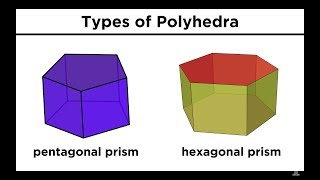14 Three-Dimensional Shapes Part 1: Types, Calculating Surface Area
Three-Dimensional Shapes Part 1: Types, Calculating Surface Area
5014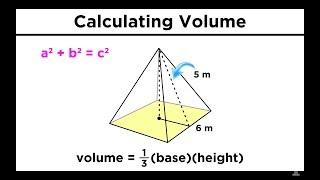15 Three-Dimensional Shapes Part 2: Calculating Volume
Three-Dimensional Shapes Part 2: Calculating Volume
5015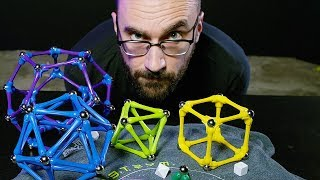Mysterium Cosmographicum
polytope, polygon, polyhedron, platonic solid, tetrahedron, cube, octahedron, dodecahedron, icosahedron
3428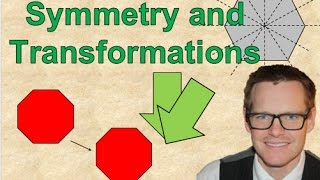Symmetry and Transformations
○ symetry, transformation, translation, rotation, reflection, dilation, congruent ○ I hope that lesson was helpful for you.
3848The History of Non-Euclidian Geometry - Sacred Geometry - Extra History - #1
The History of Non-Euclidian Geometry - Sacred Geometry - Extra History - #1
3431The History of Non-Euclidian Geometry - Squaring the Circle - Extra History - #3
The History of Non-Euclidian Geometry - Squaring the Circle - Extra History - #3
3433The History of Non-Euclidian Geometry - The Great Quest - Extra History - #2
The History of Non-Euclidian Geometry - The Great Quest - Extra History - #2
3432The History of Non-Euclidian Geometry - The World We Know - Extra History - #5
The History of Non-Euclidian Geometry - The World We Know - Extra History - #5
3435-